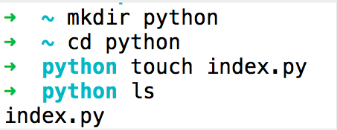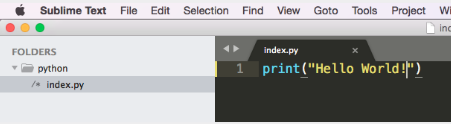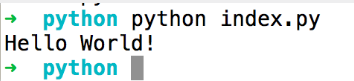# 14 Basic Features for Learning Python + Final Exercise. Part IIn this first part we are going to see the first 7 Basic Python Features:

• The print()
• The Strings
• The Variables
• The Errors
• The Numbers
• The Calculations
Python is the language par excellence for working as data scientists and for making machine learning models. In this guide we will explain the critical features to learn python in a fast, correct and enjoyable way.

Python is a programming language and like any other it serves to communicate our ideas, or the ideas of other people (the owners of the product). These ideas are the commands, which we put into a document, and this document ends up being called a "program".

Installing python is really very simple, in fact if you work in a Linux environment, it is quite likely that you already have it installed. Check this post about how to install python 3 in Linux if you don't have it yet.

Another prerequisite to work with python is to have a text editor, it can be Atom, Sublime or VisualStudio. (That's our recommended order)

First 3 steps to execute a sentence with Python2. Then open the editor of your choice and add these lines3. Now run the above code on the console.Ironically, the first thing we're going to see is how we tell the computer to ignore a part of the program

They can have these three functionalities

1. To provide context: for example, it helps to say that a variable starts at zero:
1. `var_count = 0`
2. To help others read the code: this function will help to know if it will rain tomorrow:
1. `it_will_rain()`
3. To ignore a line of code and watch the code run
1. `# usefull_value = 25`
2. `usefull_value = new_value()`

Feature #2 - The print()

In python the print() function is used to tell the computer to "talk". And the message must be surrounded by quotes

`print("My First line")`
The question here would be: are quotation marks always necessary in print?

The answer is yes, they are necessary to print strings. But they are NOT necessary to print, for example, integers, arrays of booleans.

```print("My First line")
print(10)
print(True)
print(["Daniel", "Ana"])```

Feature #3 - The Strings

We programmers refer to text blocks as "strings"

`print("My First line")`
The question here would be when to use double or single quotes?

```# Valid strings
print("Hello world")
print('we are printing with simple quotes')

# Invalid strings
print("A common use of "strings"")```
As we can see, if we open a print with single quotes we must close it anyway, the same happens with double quotes. If we need to mix both, we must "escape" the string with the syntax "\"

```# Valid strings
print("A common use of \"strings\"")```

Feature #4 - The Variables

It's the way we store information and then reuse it. The value is assigned with the sign =

```my_message = "Hello there"
print(my_message)```
It is not a coincidence that we call them variables, since they can change during the execution of the program

```my_message = "Hello there"
print(my_message)

my_message = "Hola a todos"
print(my_message)```
One question that might arise is: Can they be reassigned to another type of data? The answer is YES, as long as it is a valid data type.

```# This variable is initially assigned as a string
my_var = "Hola a todos"

# It can be reassigned to another value, regardless of the type
my_var = 85
my_var = True```

Feature #5 - The Errors

When we encounter an unexpected error, we call this "bugs".
Two common errors in python are SyntaxError and NameError

SyntaxError: means that something is mistyped in the program, such as scores where they don't belong, no closing parenthesis, etc.

NameError: when the python interpreter sees a word that it doesn't recognize, like an undefined variable for example.

Feature #6 - The Numbers

There are two types of numerical data in python: int as an integer and float as a decimal

Integer: whole number, no decimal points.

float: can be used for fractional representations

They can be assigned to a variable

```the_int = 4
the_float = 4.5

print(the_int + 3)
# prints 7```

Feature #7 - The Calculations

Python runs through all the math operations.

The division can fall into a special error: ZeroDivisionError. This is when you try to divide by zero.

```#Prints "500"
print(573 - 74 + 1)

#Prints "50"
print(25 * 2)

#Prints "2.0"
print(10/5)

#Prints "Error"
print(573 / 0)```

Conclusion:

If you come from another programming language and if you're new to programming, this little introduction will show you how flexible, powerful and simple the Python language is. Continue with our next entry with the other 7 Basic Python Features.